StatLect

Identity matrix

An identity matrix is a square matrix whose diagonal entries are all equal to one and whose off-diagonal entries are all equal to zero.

Identity matrices play a key role in linear algebra. In particular, their role in matrix multiplication is similar to the role played by the number 1 in the multiplication of real numbers:

• a real number remains unchanged when it is multiplied by 1;

• a matrix remains unchanged when it is multiplied by the identity matrix.Definition

The following is a formal definition.

Definition Letbe amatrix.is an identity matrix if and only ifwhenandwhen.

Thus, entries whose row indexand column indexcoincide (i.e., entries located on the main diagonal) are equal to. All the other entries are equal to.

When, there is only one entry, andExamples

Some examples of identity matrices follow.

Example Theidentity matrix is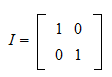Example Theidentity matrix is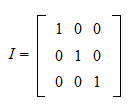Example Theidentity matrix isProducts involving the identity matrix

A key property is that a matrix remains unchanged when it is multiplied by the identity matrix.

Proposition Letbe amatrix andtheidentity matrix. Then,Proof

By the definition of matrix product, the-th entry of the productis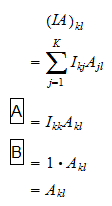where: in stepwe have used the fact thatwhen; in stepwe have used the fact that(is on the main diagonal of). Since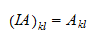for everyand,.

Proposition Letbe amatrix andtheidentity matrix. Then,Proof

The proof is similar to the previous one: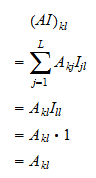The identity matrix is idempotent

A consequence of the previous two propositions is thatand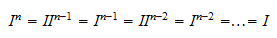In other words, any power of an identity matrix is equal to the identity matrix itself.

A matrix possessing this property (it is equal to its powers) is called idempotent.

Symmetry

Another important property of the identity matrix is that it is symmetric, that is, equal to its transpose:Proof

A matrixis symmetric if and only if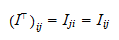for anyand. But the above equality always holds when, and it holds for identity matrices whenbecause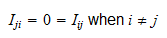The book

Most of the learning materials found on this website are now available in a traditional textbook format.

Glossary entries
Share